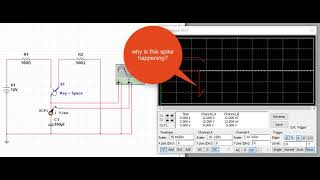# How can the current change suddenly in an RC circuit?

I was trying to simulate a circuit where a capacitor is being charged then discharged. I used an oscilloscope to measure the voltage and the current. I've noticed that when I switch the state of the capacitor from charging to discharging or from discharging to charging, the current suddenly changes to a different value.

I can understand that the voltage can change immediately, but the current? Shouldn't it have a smooth curve?

What is the explanation of what's happened with me?

What happened with me:Voltage and current measurement in an RC circuit on YouTube

• The current can change instantaneously; it's inductance that prevents that, and in an ideal RC circuit, there is no inductance. – Hearth Feb 6 at 23:34
• Can you explain why it's changing instantaneously? Shouldn't it be a smooth curve? When the voltage is increasing, the current should be decreasing, if the voltage starts to decrease, the current should increase starting from the point it's at (at least that's my understanding which is probably wrong). What's causing it to change suddenly? – StackExchange123 Feb 6 at 23:46
• To put it succinctly, $I = C·\frac{dV}{dt}$. The fact that $I$ must be finite prevents $V$ from changing instantly, but there is no such restriction on $\frac{dI}{dt}$. – Hearth Feb 7 at 0:00
• Well, looks like I have to study calculus and then take the electricity and magnetism course that I've skipped in order to fully understand. Thanks! – StackExchange123 Feb 7 at 0:06
• Current can change instantly through a capacitor. – DKNguyen Feb 7 at 2:24

I can understand that the voltage can change immediately, but the current?

• When the capacitor is charged there is 12 V on it.
• When you switch to the discharge resistor you have 12 V across 500 Ω. You should expect an immediate 24 mA to flow and this will decrease as explained by the RC discharge curve.
• When the capacitor is full discharged it will (initally) appear like a short-circuit to ground. As soon as you switch on the charge 24 mA will flow into the capacitor.

Shouldn't it have a smooth curve?

No, the simulator is correct.

Think of a cap as a tiny battery. Initially "battery" is a 0V level. So you have just connected 12V to 0V potential through the resistor. So you have a current of 12V-0V (voltage difference) over 500 ohm, as pointed out above. As the current charges the capacitor up, the voltage difference gets smaller, and the current falls too - Ohm's law still applies. Smaller voltage difference - smaller current.

Exactly identical logic for discharging. You connect 12V "battery" (capacitor) via resistor to 0V, so you have current. Which falls as the "battery" voltage falls.

Capacitors resist changes in voltage, not changes in current (that is the inductor's role).